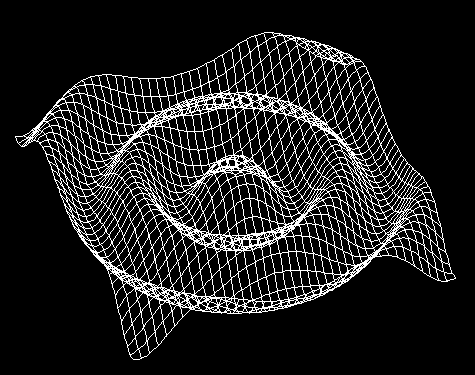# 3dimensional wave propogation.

I thought the maths area would be the best place to ask..

What kind of function would represent a 3 dimensional sine wave?
A sine wave, where the z-axis lays on the circumference of a circle.

berkeman
Mentor
I thought the maths area would be the best place to ask..

What kind of function would represent a 3 dimensional sine wave?
A sine wave, where the z-axis lays on the circumference of a circle.

What is the context of the question? The equation for a symmetric longitudinal wave in 3-D is straightforward, I think. But I'm not sure there is a solution for symmetric transverse waves in 3-D...

Essentially, the function for this:There isn't really a context, I'm not currently studying anything relating to this, it just interests me to see the behaviour of waves.

I seem to have found it, by looking for an example image.
z = sinx(√(x2+y2))

berkeman
Mentor
Essentially, the function for this:There isn't really a context, I'm not currently studying anything relating to this, it just interests me to see the behaviour of waves.

I seem to have found it, by looking for an example image.
z = sin($\sqrt{x^{2}+y^{2}}$)

Oh, I misunderstood your question then. I thought you wanted it to be symmetric in 3 dimensions, not just 2.

Oh, I misunderstood your question then. I thought you wanted it to be symmetric in 3 dimensions, not just 2.

Actually, that would be interesting..

Thank you for the assistance though. ^_^

Just solve the wave equation in three dimensions, if you are only interested in isotropic propogation then set all the angular derivatives to zero.

jasonRF
Gold Member
Just solve the wave equation in three dimensions, if you are only interested in isotropic propogation then set all the angular derivatives to zero.

I think like others I am not certain what the OP really meant, but I interpreted the question this way too - perhaps because I have a general interest in waves. Anyway, an example of a 3D plane wave would be:
$$f(x,y,z,t) = \sin\left(k_x x + k_y y + k_z z - \omega t \right)$$

olivermsun